# A 25.00 gram sample of an unknown metal initially at 99.0 degrees Celsius is added to...

A 25.00 gram sample of an unknown metal initially at 99.0 degrees Celsius is added to 50.00 grams of water initially at 12.16 degrees Celsius. The final temperature of the metal and water is 20.15 degrees Celsius. Calculate the specific heat of the metal. (The specific heat of water is 4.184 J/g*C).

(HINTS: Recall that the q of a system is equal to the -q of the surroundings. So you can set up two equations here: one for the loss of heat from the metal (the system), and one for the gain of heat by the water (the surroundings). Then substitute into one equation the variables from the other equation as appropriate. Additionally, the final temperature of the metal and the water must be the same temperature because the metal is in the water when they reach a final temperature.)

Given,

Mass of unknown sample = 25.00 g

Initial Temperature of unknown metal = 99.0C

Mass of water = 50.00 g

Initial Temperature of water = 12.16C

Final temperature of both = 20.15C

Specific heat of water = 4.184 J/g.C

Specific heat of unkown metal = ?

We known that,

Heat = m * s *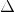T

where, m = mass

s = specific heatT = change in temperature (Final - initial)

Change in Temperature of water = 20.15C - 12.16C = 7.99C

Change in Temperature of unkown metal = 20.15C - 99.0C = -78.85C

Now,

there is not heat loss, So, Heat released by metal is absorbed by water.

- Heatm = Heatw

mm * sm *Tm = mw * sw *Tw

Put the values,

- (25.00 g * sm * -78.85C) = 50.00 g * 4.184 J/g.C * 7.99C

sm = (50.00 g * 4.184 J/g.C * 7.99C) /[-(25.00 g * -78.85C)]

sm = 0.848 J/g.C [Answer]

#### Earn Coin

Coins can be redeemed for fabulous gifts.

Similar Homework Help Questions
• ### A 25.00 gram sample of an unknown metal initially at 99.0 degrees Celsius is added to...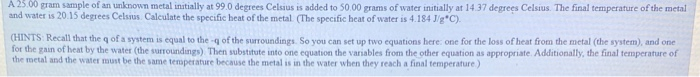A 25.00 gram sample of an unknown metal initially at 99.0 degrees Celsius is added to 50.00 grams of water initially at 14.37 degrees Celsius. The final temperature of the metal and water is 20.15 degrees Celsius Calculate the specific heat of the metal (The specific heat of water is 4.184J'g'C). (HINTS Recall that the qof a system is equal to the of the surroundings So you can set up two equations here one for the loss of heat from...

• ### 1. An unknown sample is being evaluated in lab. What is the specific heat of the...

1. An unknown sample is being evaluated in lab. What is the specific heat of the compound if it requires 105.06 J to raise the temperature of 51.68 grams of the unknown from 15.25 °C to 22.9 °C. 2. A 25.00 gram sample of an unknown metal initially at 99.0 degrees Celsius is added to 50.00 grams of water initially at 5.94 degrees Celsius. The final temperature of the system is 20.15 degrees Celsius. Calculate the specific heat of the...

• ### 1. An unknown sample is being evaluated in lab. What is the specific heat of the...

1. An unknown sample is being evaluated in lab. What is the specific heat of the compound if it requires 105.06 J to raise the temperature of 51.68 grams of the unknown from 15.25 °C to 22.9 °C. 2. A 25.00 gram sample of an unknown metal initially at 99.0 degrees Celsius is added to 50.00 grams of water initially at 5.94 degrees Celsius. The final temperature of the system is 20.15 degrees Celsius. Calculate the specific heat of the...

• ### 8 A 44.0 g sample of an unknown metal at 99.0°C was placed in a constant...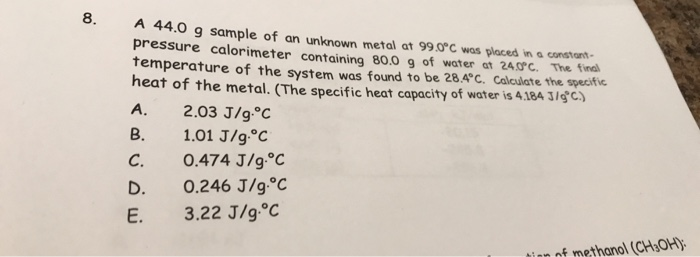8 A 44.0 g sample of an unknown metal at 99.0°C was placed in a constant pressure calorimeter containing 80.0 g of water at 24.0°c. The final temperature of the system was found to be 28.4°C. Calculate the specific heat of the metal. (The specific heat capacity of water is 4.184 J/C) 2.03 J/g C A. В. 1.01 J/g°C 0.474 J/g°C 0.246 J/g.°C 3.22 J/g.°C C. D. Е. of methanol (CH OH)

• ### a 100.0 gram metal sample is heated to a temperature of 110 degrees celcius then placed into a calorimeter containing 100.0 r of pure water (at initial temperature of 23 degrees celcius)

a 100.0 gram metal sample is heated to a temperature of 110 degrees celcius then placed into a calorimeter containing 100.0 r of pure water (at initial temperature of 23 degrees celcius). after the sample and water reach thermal equalibrium, their final temperature is 29 degrees celsius. what is the specific heat capacity of the metal sample in calories/g*degrees celsius.

• ### an ice cube at 0.00 degrees celsius with a mass of 4.52 g is placed into...

an ice cube at 0.00 degrees celsius with a mass of 4.52 g is placed into 55g of water, initially at 23 degrees celsius. If no heat is lost to the surroundings, what is the final temperature of the entire water sample after all the ice is melted? (Specific heat of water is 4.184J/g*degrees celsius)

• ### A metal sample weighing 24.000 g is heated to 100.0 degrees celsius and then transferred into a calorimeter containing 3...

A metal sample weighing 24.000 g is heated to 100.0 degrees celsius and then transferred into a calorimeter containing 30.0 mL of water at a temperature of 22.8 degrees celsius. If the specific heat of the metal is 0.105 J/g*C, what is the final temperature of the metal sample plus water?

• ### 7. A piece of metal weighing 90.3 grams and at an initial temperature of 99.0°C was...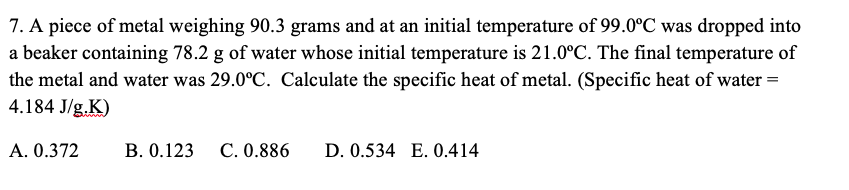7. A piece of metal weighing 90.3 grams and at an initial temperature of 99.0°C was dropped into a beaker containing 78.2 g of water whose initial temperature is 21.0°C. The final temperature of the metal and water was 29.0°C. Calculate the specific heat of metal. (Specific heat of water = 4.184 J/g.K) A. 0.372 B. 0.123 C. 0.886 D. 0.534 E. 0.414

• ### A 200 g piece of ice at 0 degrees Celsius is place in 500 g of...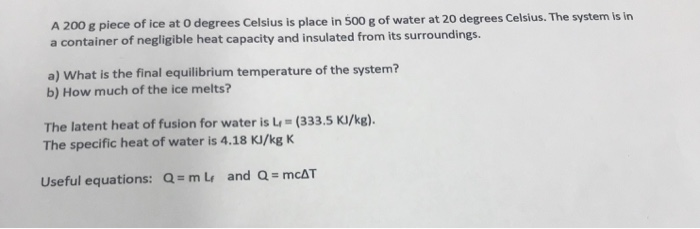A 200 g piece of ice at 0 degrees Celsius is place in 500 g of water at 20 degrees Celsius. The system is in a container of negligible heat capacity and insulated from its surroundings. a) What is the final equilibrium temperature of the system? b) How much of the ice melts? The latent heat of fusion for water is (333.5 KI/kg). The specific heat of water is 4.18 KI/kg K Useful equations: Q = mlf and Q =...

• ### a 20.94-g sample of an unknown metal is heated to 99.4 degrees Celsius in a hot water bath until thermal equilibrium is reached

a 20.94-g sample of an unknown metal is heated to 99.4 degrees Celsius in a hot water bath until thermal equilibrium is reached. The metal is quickly transferred to 100 mL of water at 22.0 degrees Celsius contained in a styrofoam cup. The thermal equilibrium temperature of the metal plus water mixture is 24.6 degrees Celsius. What is the Specific heat capacity of the metal?

Free Homework App